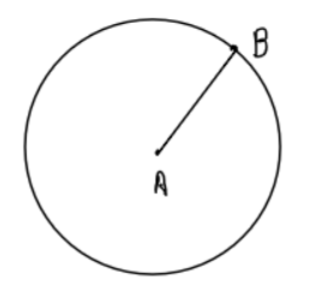Courses
Courses for Kids
Free study material
Free LIVE classes
MoreLIVE
Join Vedantu’s FREE Mastercalss

# Take two points A and B on the page of your notebook. Draw a circle with centerA which passes through B.Verified
366.6k+ views
Hint: Centre is A & radius is line segment AB.Now we have to take two points A and B such that a circle is to be drawn which passes
through B and has a center at A.
If a circle passes through a point B such that A is the center of the circle then the length AB is
surely the radius of the circle.
So, we simply need to draw a circle of radius AB. Now we are not given with any distance
hence let’s take any value of AB.
Step1 - Mark points A & B on your page.
Step 2 - Now we have to use a compass to draw the circle with centre A & radius length AB,
so place the needle of the compass on A & nib of pencil on B.
Step3 - Rotate the pencil about the needle of the compass in any direction.
Step4 - End on the point from where you started to rotate.
Note- Whenever we are given that a circle passes through a point and if the center of the
circle is also known to us then the distance between these two points is always the radius of
that circle.
Last updated date: 28th Sep 2023
Total views: 366.6k
Views today: 9.66k